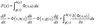# Differential equation for changing mass of a sphere

• Hypatio

#### Hypatio

The mass of a sphere with density as a function of radius is

$M=4\pi \int_0^r\rho(r) r^2dr$

Lets say the radius increases and decreases as a function of time t. So:

$M(t)=4\pi \int_{0}^{r(t)}\rho (r) r(t)^2dr$

I want to know the basic equation describing the mass added or removed from the sphere (mass increases when radius increases, mass decreases when radius decreases) as a function of t, starting from any t. The problem is I think I must use a differential form but I'm not sure what it looks like. What then is the differential form of dM(t)/dt? I think I must use a chain rule and write:

$\frac{dM}{dt}=\frac{dM}{dr}\frac{dr}{dt}$

is this right? How do I proceed to solve this with the integral?

The mass of a sphere with density as a function of radius is
$M=4\pi \int_0^r\rho(r) r^2dr$
Lets say the radius increases and decreases as a function of time t. So:
$M(t)=4\pi \int_{0}^{r(t)}\rho (r) r(t)^2dr$

Hi !
I am afraid that there is something wrong in your writting. It should be :
$M=4\pi \int_0^r\rho(u) u^2du$
Lets say the radius increases and decreases as a function of time t. So:
$M(t)=4\pi \int_{0}^{r(t)}\rho (u) u^2du$
You may use any other symbol than u, but not r.

Ah yes, sure, the upper limit is the 'full' radius (r) and u is a radius. This doesn't solve my problem though.

Yes, the chain rule: $\dfrac{dM}{dt}= \dfrac{dM}{dr}\dfrac{dr}{dt}$
JJaquelines point helps make sense of the dM/dr.

To find $\dfrac{dM}{dt}$ use the "fundamental theorem of Calculus":
$$\frac{d}{dr}\int_0^r \rho(u)u^2 du= \rho(r)r^2$$

I guess the solution then is

$\frac{dM}{dt}=\frac{4}{3}\pi \rho(r)r^3\frac{dr}{dt}$

does the solution change if $\rho(r)$ becomes $\rho(r,t)$ or could I write

$\frac{dM}{dt}=\frac{4}{3}\pi \rho(r,t)r^3\frac{dr}{dt}$

Thanks.

Last edited:
I don't think so. A term involving the partial derivatine of rho relatively to t is missing into your last equation,

Is there a rule I can apply to get this additional term?

Is there a rule I can apply to get this additional term?

If you have
$$F(t) = \int_0^{a(t)} g(r,t)\,\mathrm{d}r$$
then
$$F'(t) = \int_0^{a(t)} \left.\frac{\partial g}{\partial t}\right|_{(r,t)}\,\mathrm{d}r + a'(t)g(a(t),t)$$
assuming $g$ is sufficiently smooth.

Please do not mix together dummy variables in the integrand with integral limits.
Properly speaking, you have the following the relation:
$$M(t)=4\pi\int_{0}^{r(t)}\rho(x)x^{2}dx$$
Thus, you have:
$$\frac{dM}{dt}=4\pi\rho(r(t))r(t)^{2}\frac{dr}{dt}$$
which has as interpretation that only the outermost spherical shell at r(t) determines the total change of mass.

Every compact ball strictly contained within the outermost shell (radii less than r(t)) remains constant in mass.

However:
Suppose you have a ball where at different times, the density at some fixed radius "x" may change as a function of time. Then, you have:
$$M(t)=4\pi\int_{0}^{r(t)}\rho(x,t)x^{2}dx$$
In this case, the total mass of the ball will be due to two distinct effects:
1. The ball shrinks or expands. This gives the contribution given above.
2. The interior of the ball may change its mass. This effect is new.

In sum, you'll then get:
$$\frac{dM}{dt}=4\pi\rho(r(t),t)r(t)^{2}\frac{dr}{dt}+4\pi\int_{0}^{r(t)}\frac{\partial\rho}{\partial{t}}x^{2}dx$$

Last edited:
•1 person
Is there a rule I can apply to get this additional term?
The general formula below shows the rule for derivation :

#### Attachments

•Derivation rule.JPG
6 KB · Views: 406
•1 person
Hypatio said:
So the chain rule no longer applies??
Of course, the chain rule continues to applies !
The formula given above is the application of the chain rule in case of an integral with the integrand and limits which are functions of the variable considered for derivation.